# Quotient Identities

There are two quotient identities that can be used in right triangle trigonometry. A quotient identity defines the relations for tangent and cotangent in terms of sine and cosine.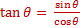and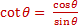Let's look at the tangent quotient identity using a familiar 30-60-90 triangle.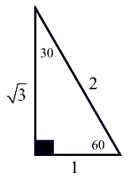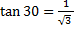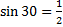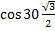Substitute the appropriate values into the identity and simplify: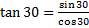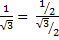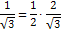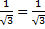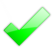Let's look at the cotangent quotient identity using a familiar 45-45-90 triangle.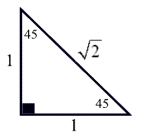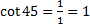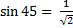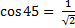Substitute the appropriate values into the identity and simplify: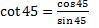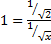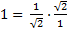1 = 1The quotient identities will be used in trigonometric proofs and applications of calculus where using an identity is a more convenient form. Remember that the difference between an equation and an identity is that an identity will be true for ALL values.

 Related Links: Math Trigonometry Pythagorean Identities Cofunction Identities

To link to this Quotient Identities page, copy the following code to your site: